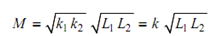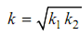## Coefficient of Coupling Assignment Help

Assignment Help: >> ElectroMagnetism - Coefficient of Coupling

Coefficient of Coupling:

While two coils are coupled magnetically, it is significant to know how much of the flux generated by one coil is linking with the other. Coefficient of coupling gives an idea about that.

Let us consider again the magnetically coupled coils of Figure Following Eq. (9), inductance of coil1 and coil 2 may be written as :

L1 = N1  (φ1 /i1 )

L 2= N2   (φ2  /i2 )

Let Φ1 be the total flux produced by current i1 in coil 1 and Φ12 be the part of Φ1 that is linked with coil 2.

Let                                             φ12 = k1 φ1

From Eq. (20),

M = N (φ12 /i12 )= N k1 φ1  /i

Likewise, let Φ2 be the total flux produced by current i2 in coil 2 and Φ21 be the part of Φ2 that is linked with coil1.

Let                       φ21  = k2 φ2

From Eq. (2.21),

M = N (φ21 /i12 ) = N1( k2 φ2/ i12)

Multiplying Eqs. (24) and (25), we get,

M 2  = k1 k2 N1 N2 φ1 φ2 / i1i2= k1 k2  =(  N1 φ1 / i12)( N2 φ2 / i12 )= k1 k2  L1 L2where ∴So, From Eq. (26), we obtain,Constant k is called as coefficient of coupling and this is defined as the ratio of mutual inductance M to the square root of the product of inductances of coil 1 and coil 2.

If k =1, you know that the flux because of one coil is fully connected with the other. If k = 0, the flux in one coil does not link with the other coil at all.#### Assured A++ Grade

Get guaranteed satisfaction & time on delivery in every assignment order you paid with us! We ensure premium quality solution document along with free turntin report!

All rights reserved! Copyrights ©2019-2020 ExpertsMind IT Educational Pvt Ltd